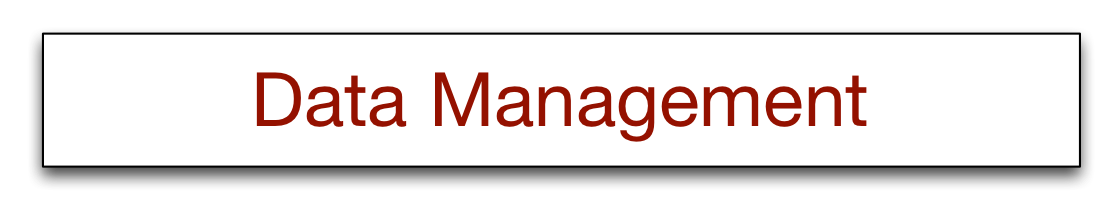# 16 - Data Visualization Slide Exercises

Create a point plot with a square shape using the data in the following table.
 Year 1804 1927 1960 1974 1987 1999 2012 2027 2046 Population (billions) 1 2 3 4 5 6 7 8 9
`library(ggvis)library(tibble)year <-  c(1804,1927,1960,1974,1987,1999,2012,2027,2046)pop <-  (1:9)df <-  tibble(year,pop)df %>%   ggvis(~year, ~pop) %>%   layer_points(fill:="green",shape:="square" ) %>%  add_axis('x', title = 'Year', format='####', subdivide=3, values = seq(1800, 2050, by = 50)) %>%  add_axis('y',title='Population')`
The global average cost of solar panels dropped from \$9.70 per watt in 1980 to \$3.03 per watt in 2005, and further dropped to 75 cents per watt in 2015. Create a line plot.
`library(ggvis)library(tibble)year <-  c(1980,2005,2015)price <-  c(9.7,3.03,.75)df <-  tibble(year,price)df %>% ggvis(~year, ~price) %>%   layer_lines(stroke:= 'green') %>%   add_axis('x', title = 'Year', format='####', subdivide=1, values = seq(1970, 2020, by = 10)) %>%  add_axis('y',title='\$ per watt')`

Create a bar chart using the data in the following table.
 Year 1804 1927 1960 1974 1987 1999 2012 2027 2046 Population (billions) 1 2 3 4 5 6 7 8 9
`library(ggvis)library(tibble)year <-  c(1804,1927,1960,1974,1987,1999,2012,2027,2046)pop <-  (1:9)df <-  tibble(year,pop)df %>%   ggvis( ~year, ~pop) %>%   layer_bars(fill:='salmon') %>%  add_axis('x', title = 'Year',  format='####', subdivide=1, values = seq(1800, 2050, by = 50)) %>%  add_axis('y',title='Population')`

National GDP and fertility data have been extracted from a web site and saved as a CSV file.
Compute the correlation between GDP and fertility.
Do a scatterplot of GDP versus fertility with a smoother.
Log transform both GDP and fertility and repeat the scatterplot with a smoother.

```# GDP & fertilitylibrary(ggvis)# source http://www.indexmundi.com/g/correlation.aspx?v1=67&v2=31&y=2004d <- read.table('http://people.terry.uga.edu/rwatson/data/gdp&fertility.csv',header=T,sep=',')
cor.test(d\$GDP,d\$Fertility)
d %>%
ggvis(~GDP, ~Fertility) %>%
layer_points(fill:='tomato') %>%
layer_smooths(stroke:='darkblue')
d %>% ggvis(~GDP, ~Fertility) %>%
mutate(GDP = log(GDP)) %>%
mutate(Fertility = log(Fertility)) %>%
layer_points(fill:='tomato') %>%
layer_smooths(stroke:='darkblue') %>%